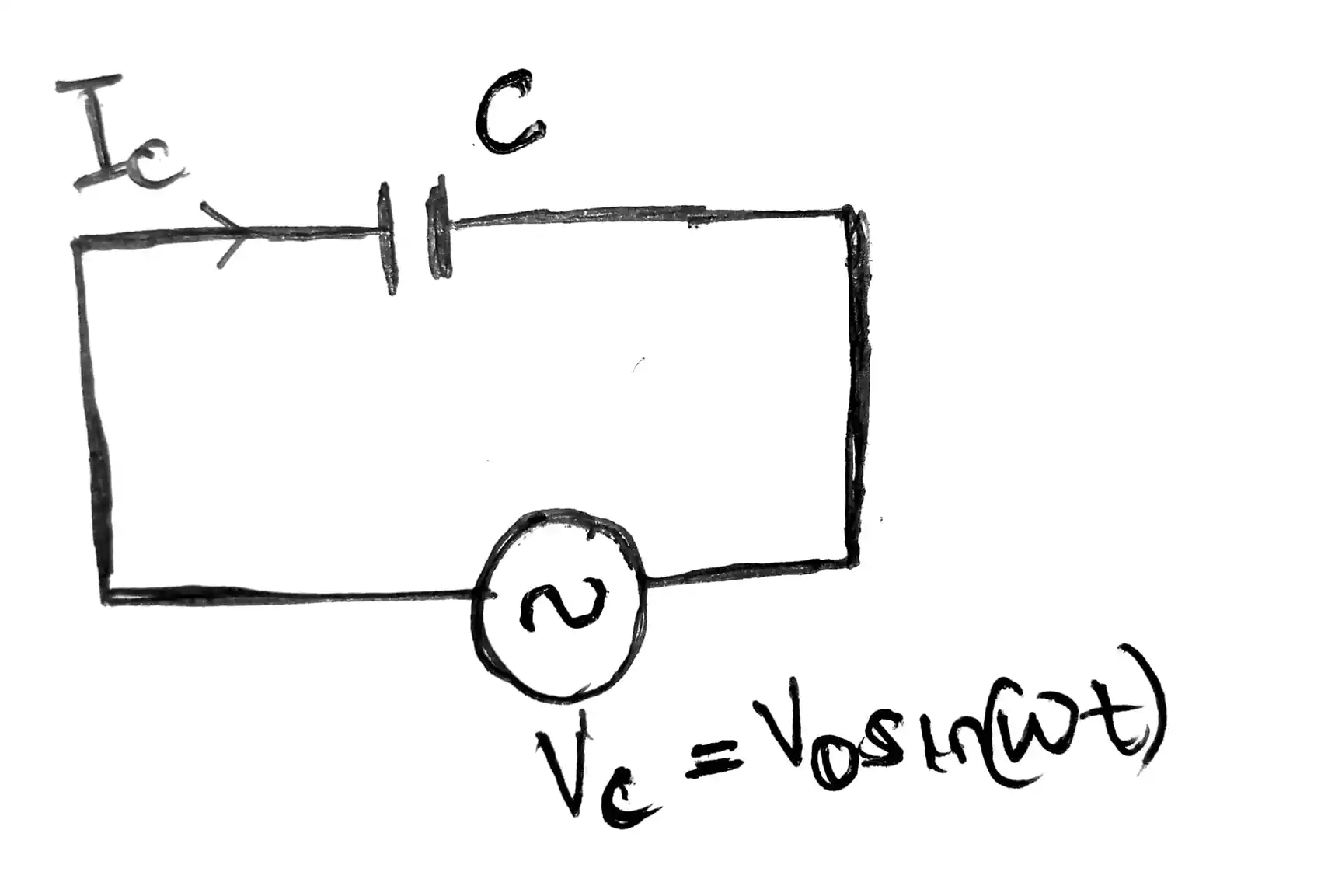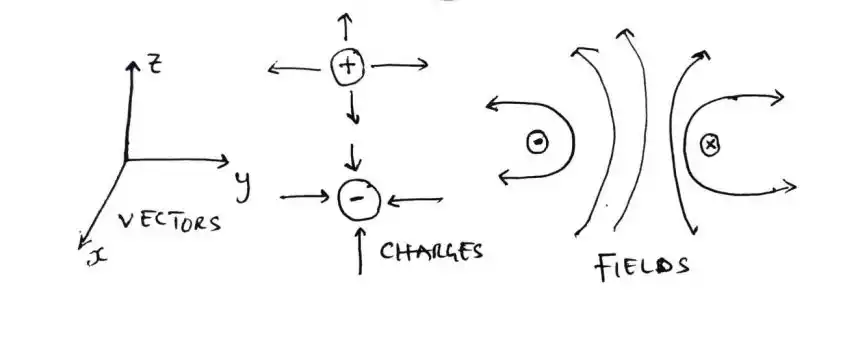# What is the Importance of Maxwell's Equations in Electromagnetism?

Maxwell’s equations, formulated by James Clerk Maxwell in the mid-19th century, represent the cornerstone of classical electromagnetism, classical optics, and electric circuits.

These four partial differential equations elegantly describe the behavior of electric and magnetic fields and have far-reaching implications across multiple scientific disciplines.

In this comprehensive article, we look into the crucial importance of Maxwell’s equations in electromagnetism and explore their profound impact on technology, research, and everyday life.

## The Four Maxwell Equations – Foundation of Electromagnetism

### 1. Gauss’s Law for Electric Field

This equation reveals a profound connection between electric charges and electric fields.

It states that the divergence of the electric field is equal to the electric charge density.

Mathematically, it’s expressed as ∇ · E = ρ / ε₀, where;

• ∇ – denotes the divergence operator,
• E – represents the electric field,
• ρ – is the electric charge density, and
• ε₀ – is the permittivity of free space.

### 2. Gauss’s Law for Magnetism

Unlike electric monopoles, magnetic monopoles have never been observed. Gauss’s law for magnetism reflects this by stating that the divergence of the magnetic field is always zero (∇ · B = 0).

This equation helps explain why magnetic field lines always form closed loops.

### 3. Faraday’s Law of Induction

This equation demonstrates how changing magnetic fields induce electric fields.

It quantifies the curl of the electric field, showing that it’s equal to the negative of the time derivative of the magnetic field (∇ × E = -∂B/∂t).

Faraday’s law underlies the operation of generators and transformers, pivotal devices in our power infrastructure.

### 4. Ampère’s Law with Maxwell’s Addition

Ampère’s law describes the relationship between electric currents and magnetic fields.

Maxwell’s addition introduces a new term—the displacement current—to the equation, accounting for changing electric fields.

This modified Ampère’s law (∇ × B = μ₀J + μ₀ε₀∂E/∂t) ensures the consistency of electromagnetic theory.

## The Significance of Maxwell’s Equations

Maxwell’s equations have a significance that reaches far beyond theoretical physics.

They hold the key to understanding various natural phenomena and technological occurrences that shape our modern world.

Here’s why they matter:

1. Prediction of Electromagnetic Waves: Perhaps one of the most groundbreaking implications of Maxwell’s equations is the prediction of electromagnetic waves.

These equations describe how changing electric and magnetic fields propagate through space, leading to the discovery of radio waves, microwaves, X-rays, and more.

The realization that light itself is an electromagnetic wave was a transformative moment in our understanding of the universe.
2. Technological Innovations: Maxwell’s equations have been a driving force behind numerous technological innovations.

From radio and television to radar and lasers, these equations underpin the functionality of countless devices we rely on daily.

For instance, radio communication wouldn’t be possible without a deep understanding of how electromagnetic waves travel through space.
3. Medical Imaging: Maxwell’s equations find application in medical imaging techniques such as MRI (Magnetic Resonance Imaging).

MRI machines use strong magnetic fields to create detailed images of internal body structures, revolutionizing diagnosis and treatment in the medical field.
4. Telecommunications: The efficient transmission and reception of signals in telecommunications systems are made possible by antennas designed using Maxwell’s equations.

These antennas ensure the accurate propagation of radio, television, and cellular signals, connecting the world like never before.
5. Optical Device Design: Maxwell’s equations extend their influence to optics.

They aid in the design of lenses, mirrors, and other optical components, enabling technologies like cameras, telescopes, and microscopes.

## How Maxwell’s Equations are Used in Practice

To grasp the tangible impact of Maxwell’s equations, consider these practical applications:

1. Calculating Fields: Engineers and scientists employ Maxwell’s equations to calculate electric and magnetic fields around charged particles and current-carrying wires.

This knowledge is vital for designing circuits, electronic devices, and systems.
2. Wave Behavior Prediction: Maxwell’s equations enable accurate predictions of how electromagnetic waves behave.

This insight is pivotal for designing wireless communication systems and optimizing signal transmission.
3. Antenna Design: Antennas used in devices like smartphones, satellite dishes, and Wi-Fi routers rely on Maxwell’s equations for their design.

These equations guide engineers in creating antennas that efficiently transmit and receive electromagnetic signals.
4. Media Interaction Analysis: Researchers use Maxwell’s equations to analyze how electromagnetic waves interact with different materials.

This knowledge is vital for developing materials that absorb or reflect specific wavelengths, with applications in energy-efficient buildings and stealth technology.
5. Optical System Development: Maxwell’s equations play a crucial role in designing optical systems, including eyeglasses, microscopes, and lasers.

This ensures precise control over light propagation and manipulation.

## Wrap Up

Maxwell’s equations stand as an enduring testament to the power of scientific insight and human ingenuity.

These equations, which describe the fundamental nature of electric and magnetic fields, have paved the way for technological advancements that have transformed our lives.

From enabling wireless communication to enhancing medical diagnostics, Maxwell’s equations continue to shape our understanding of the physical world and drive innovations that propel us into the future.

## FAQs

1. What Are Maxwell’s Equations? Maxwell’s equations are a set of four partial differential equations that describe the behavior of electric and magnetic fields.

They were formulated by James Clerk Maxwell and are fundamental to understanding electromagnetism.
2. Why Are Maxwell’s Equations Important? Maxwell’s equations underpin our comprehension of electromagnetism, enabling the prediction of electromagnetic waves and the design of technologies like radios, antennas, and medical imaging devices.
3. How Do Maxwell’s Equations Impact Technology? These equations drive technological innovation, shaping everything from communication systems (radio, television) to medical diagnostics (MRI) and optical devices (lenses, lasers).
4. How Do Maxwell’s Equations Influence Optical Design? Optical devices like lenses, mirrors, and cameras rely on Maxwell’s equations for precise design.

They guide the manipulation of light, enabling advancements in fields like photography and microscopy.
5. What Role Do Maxwell’s Equations Play in Antenna Design? Maxwell’s equations inform the design of antennas used in modern devices, such as smartphones and satellite dishes.

These equations ensure efficient transmission and reception of signals.Solved: Verify that the conduction current in the wire equals the displacement current ...Resources to help you learn and understand Electromagnetics field theory from scratch10 Important facts you need to know about magnets and magnetic fieldsThe Easiest Way to Study Electromagnetics Theory